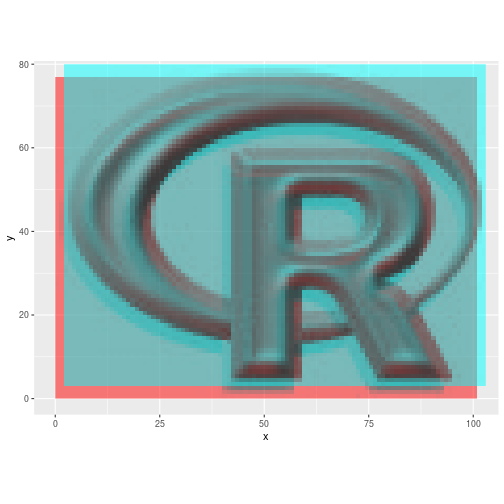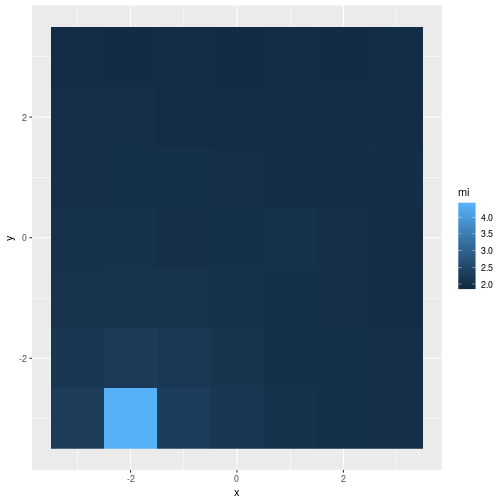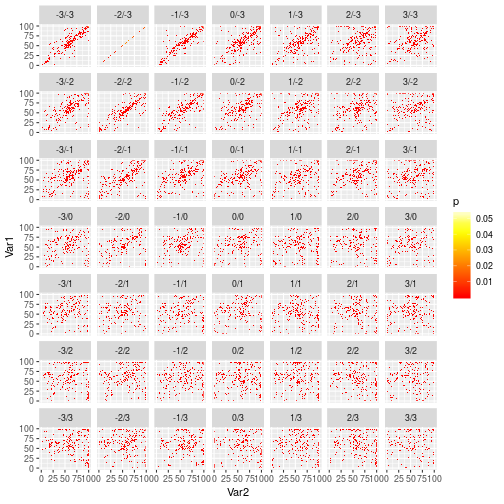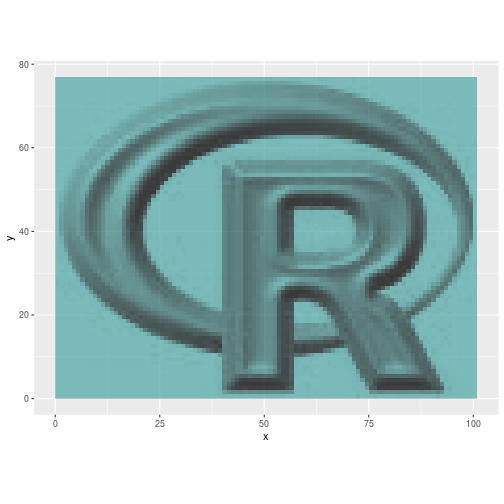coregisterImages { RStoolbox} R Documentation

### Image to Image Co-Registration based on Mutual Information

R: Image to Image Co-Registration based on Mutual Information

### Description

Shifts a slave image to match the reference image (master). Match is based on maximum mutual information.

### Usage

```coregisterImages(slave, master, shift = 3, shiftInc = 1,
nSamples = 1e+05, reportStats = FALSE, verbose, nBins = 100, ...)
```

### Arguments

 `slave` Raster* object. Slave image to shift to master. Slave and master must have equal numbers of bands. `master` Raster* object. Reference image. Slave and master must have equal numbers of bands. `shift` Numeric or matrix. If numeric, then shift is the maximal absolute radius (in pixels of `slave` resolution) which `slave` is shifted (`seq(-shift, shift, by=shiftInc)`). If shift is a matrix it must have two columns (x shift and y shift), then only these shift values will be tested. `shiftInc` Numeric. Shift increment (in pixels, but not restricted to integer). Ignored if `shift` is a matrix. `nSamples` Integer. Number of samples to calculate mutual information. `reportStats` Logical. If `FALSE` it will return only the shifted images. Otherwise it will return the shifted image in a list containing stats such as mutual information per shift and joint histograms. `verbose` Logical. Print status messages. Overrides global RStoolbox.verbose option. `nBins` Integer. Number of bins to calculate joint histogram. `...` further arguments passed to `writeRaster`.

### Details

Currently only a simple linear x - y shift is considered and tested. No higher order shifts (e.g. rotation, non-linear transformation) are performed. This means that your imagery should already be properly geometrically corrected.

Mutual information is a similarity metric originating from information theory. Roughly speaking, the higher the mutual information of two data-sets, the higher is their shared information content, i.e. their similarity. When two images are exactly co-registered their mutual information is maximal. By trying different image shifts, we aim to find the best overlap which maximises the mutual information.

### Value

`reportStats=FALSE` returns a Raster* object (x-y shifted slave image). `reportStats=TRUE` returns a list containing a data.frame with mutual information per shift (\$MI), the shift of maximum MI (\$bestShift), the joint histograms per shift in a list (\$jointHist) and the shifted image (\$coregImg).

### Examples

``````library(raster)
library(ggplot2)
library(reshape2)
data(rlogo)
reference <- rlogo
## Shift reference 2 pixels to the right and 3 up
missreg <- shift(reference,  2,  3)

## Compare shift
p <- ggR(reference, sat = 1, alpha = .5)
p + ggR(missreg, sat = 1, hue = .5, alpha = 0.5, ggLayer=TRUE)
````````````## Coregister images (and report statistics)
coreg <- coregisterImages(missreg, master = reference,
nSamples = 500, reportStats = TRUE)

## Plot mutual information per shift
ggplot(coreg\$MI) + geom_raster(aes(x,y,fill=mi))
````````````## Plot joint histograms per shift (x/y shift in facet labels)
## No test:

df <- melt(coreg\$jointHist)
df\$L1 <- factor(df\$L1, levels = names(coreg\$jointHist))
df[df\$value == 0, "value"] <- NA ## don't display p = 0
ggplot(df) + geom_raster(aes(x = Var2, y = Var1,fill=value)) + facet_wrap(~L1) +
scale_fill_gradientn(name = "p", colours =  heat.colors(10), na.value = NA)
``````
``````#> Warning: Removed 438677 rows containing missing values (geom_raster).
````````````## End(No test)
## Compare correction
ggR(reference, sat = 1, alpha = .5) +
ggR(coreg\$coregImg, sat = 1, hue = .5, alpha = 0.5, ggLayer=TRUE)
``````[Package RStoolbox version 0.2.6 Index]